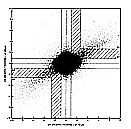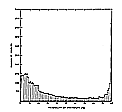VII.D.3 Variable Sources

# IRAS Explanatory Supplement VII. Analysis of Processing D. Photometric Accuracy D.3 Variable Sources

Chapter Contents | Introduction | Authors | ReferencesFigure VII.D.9 HCON-to-HCON flux variations at 12 and 25  µm, normalized with respect to the resultant HCON uncertainty. larger largest
The method by which a probability of variability as quotedin the catalog is calculated for a source with 12 and 25  µm fluxes is given in Section V.H.5. Figure VII.D.9 shows the distribution of the flux density variations between HCONs in units of the standard deviation for 25 versus 12 µm flux densities. Only catalog sourcesin unconfused areas of the sky are plotted. Sources in the shaded region (a) have a "probability of variability" between 0.5 and 0.99. Those sources in the outer regions (b) are all flagged with a probability of variability greater than 0.99.

Table VII.D.4 gives the approximate relative change in the flux densities at 12 and 25 µm associated with a given probability of variability. Two values are given, one for bright sources and one for faint sources. The table shows that there is a strong increase in the observed relative flux change as the quoted probability of variability increases from 90 to 99%. It is seen that variable sources whose fluxes change by 20% or more at 12 and 25 µm canbe identified reliably. A caution is necessary, however. In regions of high source density, scan-to-scan variations in the baseline can affect the measurements enough to produce spurious indications of variability.

Approximate Relative Flux Change at 12 and 25 µm
Table VII.D.4
Probability of Variability Bright SourcesFaint Sources
0.50 10% 15%
0.75 15% 20%
0.90 20% 30%
0.99 30% 40%Figure VII.D.10 A histogram showing the number of sources with a given probability of variability. larger largest

Figure VII.D.10 shows the distribution in number of sourcesvs. probability of variability quoted in the catalog. Twenty-five percent of eligible sources have a probability of variability greater than 50%; 13% have a probability of variability above 90%. Sources with a high probability of variability preferentially vary on a long time scale with roughly ten times as many sources varying over a time scale of 6 months as on time scales of weeks.

Figure VII.Ap.16 shows the distribution on the sky of sources flagged with a probability of variability of 0.9 or greater. The plot clearly shows a population of sources occupying a region near the center of the Galaxy, probably due to a population of highly variable sources in the Galactic bulge. In the Galactic plane at Galactic longitude 320°, there is a diagonal edge showing a marked increase in the density of variable sources. This, as well as other more subtle structures, is probably a sampling effect since in this region the source density is high and the survey strategy produced extra HCONs at time intervals suitable for detecting variability. This same effect is also seen in the Large Magellanic Cloud, which because it was at the south ecliptic pole received many coverages.

Chapter Contents | Introduction | Authors | References